## My Second (R) Shiny App: Sampling Distributions & CLTImage Credit: Doug Buckley of http://hyperactive.to

I was so excited about my initial foray into Shiny development using jennybc‘s amazing package that I stayed up half the night last night (again) working on my second Shiny app: a Shiny-fied version of the function I shared in March to do simulations illustrating sampling distributions and the Central Limit Theorem using many different source distributions. (Note that Cauchy doesn’t play by the rules!) Hope this info is useful to all new Shiny developers.

If the app doesn’t work for you, it’s possible that I’ve exhausted my purchased hours at http://shinyapps.io — no idea how much traffic this post might generate. So if that happens to you, please try getting Shiny to work locally, cutting and pasting the code below into server.R and ui.R files, and then launching the simulation from your R console.

Here are some important lessons I learned on my 2nd attempt at Shiny development:

• Creating a container (rv) for the server-side values that would change as a result of inputs from the UI was important. That container was then available to the portions of my Shiny code that prepared data for the UI, e.g. output\$plotSample.
• Because switch only takes arguments that are 1 character long, using radio buttons in the Shiny UI was really useful: I can map the label on each radio button to one character that will get passed into the data processing on the server side.
• I was able to modify the CSS for the page by adding a couple lines to mainPanel() in my UI.
• Although it was not mentally easy (for me) to convert from an R function to a Shiny app when initially presented with the problem, in retrospect, it was indeed straightforward. All I had to do was take the original function, split out the data processing from the presentation (par & hist commands), put the data processing code on the server side and the presentation code on the UI side, change the variable names on the server side so that they had the input\$ prefix, and make sure the variable names were consistent between server and UI.
• I originally tried writing one app.R file, but http://shinyapps.io did not seem to like that, so I put all the code that was not UI into the server side and tried deploying with server.R and ui.R, which worked. I don’t know what I did wrong.
• If you want to publish to http://shinyapps.io, the directory name that hosts your files must be at least 4 characters long or you will get a “validation error” when you attempt to deployApp().
```## Nicole's Second Shiny Demo App
## N. Radziwill, 12/6/2015, http://qualityandinnovation.com
## Used code from http://github.com/homerhanumat as a base
###########################################################
## ui
###########################################################

ui <- fluidPage(
titlePanel('Sampling Distributions and the Central Limit Theorem'),
sidebarPanel(
helpText('Choose your source distribution and number of items, n, in each
sample. 10000 replications will be run when you click "Sample Now".'),
href="http://www.r-bloggers.com/sampling-distributions-and-central-limit-theorem-in-r/", target="_blank")),
sliderInput(inputId="n","Sample Size n",value=30,min=5,max=100,step=2),
c("Exponential: Param1 = mean, Param2 = not used" = "E",
"Normal: Param1 = mean, Param2 = sd" = "N",
"Uniform: Param1 = min, Param2 = max" = "U",
"Poisson: Param1 = lambda, Param2 = not used" = "P",
"Cauchy: Param1 = location, Param2 = scale" = "C",
"Binomial: Param1 = size, Param2 = success prob" = "B",
"Gamma: Param1 = shape, Param2 = scale" = "G",
"Chi Square: Param1 = df, Param2 = ncp" = "X",
"Student t: Param1 = df, Param2 = not used" = "T")),
numericInput("param1","Parameter 1:",10),
numericInput("param2","Parameter 2:",2),
actionButton("takeSample","Sample Now")
), # end sidebarPanel
mainPanel(
# Use CSS to control the background color of the entire page
tags\$style("body {background-color: #9999aa; }")
),
plotOutput("plotSample")
) # end mainPanel
) # end UI

##############################################################
## server
##############################################################

library(shiny)
r <- 10000 # Number of replications... must be ->inf for sampling distribution!

palette(c("#E41A1C", "#377EB8", "#4DAF4A", "#984EA3",
"#FF7F00", "#FFFF33", "#A65628", "#F781BF", "#999999"))

server <- function(input, output) {
set.seed(as.numeric(Sys.time()))

# Create a reactive container for the data structures that the simulation
# will produce. The rv\$variables will be available to the sections of your
# server code that prepare output for the UI, e.g. output\$plotSample
rv <- reactiveValues(sample = NULL,
all.sums = NULL,
all.means = NULL,
all.vars = NULL)

# Note: We are giving observeEvent all the output connected to the UI actionButton.
# We can refer to input variables from our UI as input\$variablename
observeEvent(input\$takeSample,
{
my.samples <- switch(input\$src.dist,
"E" = matrix(rexp(input\$n*r,input\$param1),r),
"N" = matrix(rnorm(input\$n*r,input\$param1,input\$param2),r),
"U" = matrix(runif(input\$n*r,input\$param1,input\$param2),r),
"P" = matrix(rpois(input\$n*r,input\$param1),r),
"C" = matrix(rcauchy(input\$n*r,input\$param1,input\$param2),r),
"B" = matrix(rbinom(input\$n*r,input\$param1,input\$param2),r),
"G" = matrix(rgamma(input\$n*r,input\$param1,input\$param2),r),
"X" = matrix(rchisq(input\$n*r,input\$param1),r),
"T" = matrix(rt(input\$n*r,input\$param1),r))

# It was very important to make sure that rv contained numeric values for plotting:
rv\$sample <- as.numeric(my.samples[1,])
rv\$all.sums <- as.numeric(apply(my.samples,1,sum))
rv\$all.means <- as.numeric(apply(my.samples,1,mean))
rv\$all.vars <- as.numeric(apply(my.samples,1,var))
}
)

output\$plotSample <- renderPlot({
# Plot only when user input is submitted by clicking "Sample Now"
if (input\$takeSample) {
# Create a 2x2 plot area & leave a big space (5) at the top for title
par(mfrow=c(2,2), oma=c(0,0,5,0))
hist(rv\$sample, main="Distribution of One Sample",
ylab="Frequency",col=1)
hist(rv\$all.sums, main="Sampling Distribution of the Sum",
ylab="Frequency",col=2)
hist(rv\$all.means, main="Sampling Distribution of the Mean",
ylab="Frequency",col=3)
hist(rv\$all.vars, main="Sampling Distribution of the Variance",
ylab="Frequency",col=4)
mtext("Simulation Results", outer=TRUE, cex=3)
}
}, height=660, width=900) # end plotSample

} # end server```

## Control Charts in R: A Guide to X-Bar/R Charts in the qcc Package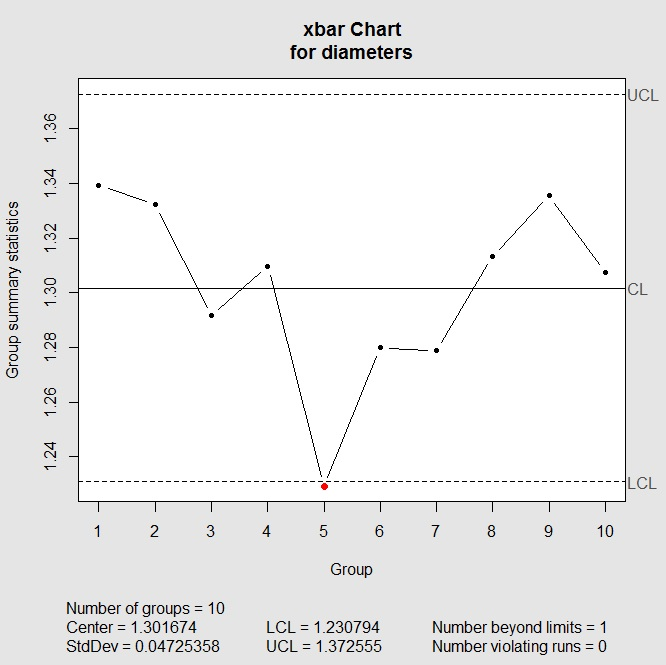Statistical process control provides a mechanism for measuring, managing, and controlling processes. There are many different flavors of control charts, but if data are readily available, the X-Bar/R approach is often used. The following PDF describes X-Bar/R charts and shows you how to create them in R and interpret the results, and uses the fantastic qcc package that was developed by Luca Scrucca. Please let me know if you find it helpful!

Creating and Interpreting X-Bar/R Charts in R

## Logistic Growth, S Curves, Bifurcations, and Lyapunov Exponents in R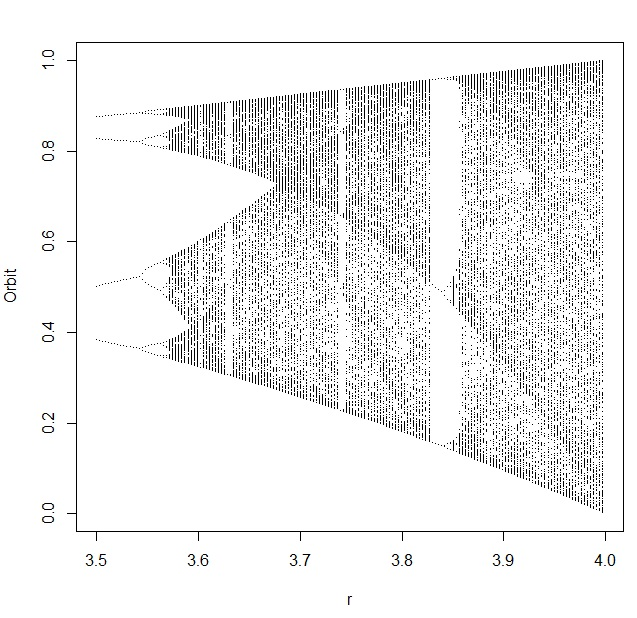If you’ve ever wondered how logistic population growth (the Verhulst model), S curves, the logistic map, bifurcation diagrams, sensitive dependence on initial conditions, “orbits”, deterministic chaos, and Lyapunov exponents are related to one another… this post explains it in just 10 steps, each with some code in R so you can explore it all yourself. I’ve included some code written by other people who have explored this problem (cited below) as portions of my own code.

It all starts with a hypothesized population… and a process where the size of the population changes over time. We want to understand how (and under what conditions) those changes occur, so we choose a model that characterizes population changes: the logistic growth model. It’s been used in biology, ecology, econometrics, marketing, and other areas.

1. The logistic growth model describes how the size of a population (N) changes over time (t), based on some maximum population growth rate (r). There is a limiting factor called the carrying capacity (K) which represents the total population that the environment could support, based on the amount of available resources. dN/dt is the rate of change of the population over time.2. You can simplify the logistic growth model by defining a new variable x to represent the portion of the population that’s alive, compared to the total population that the environment could support (and keep alive). So with x = N/K, you get a new differential equation in terms of x. Now we are looking at the rate of change of the population fraction over time. Once x = N/K = 1, the environment can’t support any more members in the population: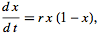3. You can solve this equation by integration! Then, you’ll have an expression that you can use to calculate x (which is still the population fraction) for any time t. This is called the sigmoid or (more commonly), the S Curve. To compute x at any time t, all we need to know is how big the population was when we started looking at it (x0) and the maximum growth rate r:4. The equation for the S Curve is deterministic and continuous. If we want to solve it numerically, we have to discretize it by chopping up that continuous axis that contains time into little tiny pieces of time. That’s what produces the difference equation that we recognize as the logistic map. It’s a map because it “maps” each value of the sequence onto the next value in the sequence. As long as you know one of those values for x (indicated by the subscript n), you’ll be able to figure out the next value of x (indicated by the subscript n+1). The value x[n] is the population fraction of the current generation, and the value x[n+1] is the population fraction for the next generation. This makes the logistic map a Markov chain. If you plot x[n] on the x axis and x[n+1] on the y axis, this expression will produce the familiar upside down parabola: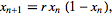5. The logistic map behaves differently depending upon the maximum growth rate (r) that describes your population. This parameter is also called fecundity and represents how rabbit-like your population is reproducing. The higher the r, the more productive, like rabbits (although I’m not sure precisely which r you’d choose if you were studying rabbits). Here is an R function that you can use to generate the last M iterations from a sequence of N total, developed and described at Mage’s Blog:

```logistic.map <- function(r, x, N, M) {
## from http://www.magesblog.com/2012/03/logistic-map-feigenbaum-diagram.html
## r: bifurcation parameter
## x: initial value, something greater than 0 and less than 1
## N: number of iterations (total)
## M: number of iteration points to be returned
z <- 1:N
z <- x
for(i in c(1:(N-1))){
z[i+1] <- r *z[i] * (1 - z[i])
}
## Return the last M iterations
z[c((N-M):N)]
}```

6. The logistic map has many interesting properties, but here are two in particular (the first in Step 6 and the second in Step 7). First, for several values you can choose for r, the chain converges to a single value (or fixed point) when n gets really big. For other values of r, the value of x will eventually bounce between two values instead of converging (a limit cycle of 2). For other values of r, the value of x will eventually bounce between four values instead of converging. Sometimes, x will bounce around a near limitless collection of values (a condition called deterministic chaos). The eventual values (or collection of eventual values, if they bounce between values) is called an orbit. For example, when the growth rate r is 2.6, the logistic map rapidly converges to an orbit of about 0.615:

`plot(logistic.map(2.6,.01,20,20), type="l")`7. Sometimes, it can be nice to take a look at how the values bounce around, and where they eventually converge (or not). To do this, we use cobweb diagrams (which are also sometimes called web diagrams). I used a function that I found at http://bayesianbiologist.com to plot the behavior of the orbits for r=2.6, r=3.2, and r=3.9:

```logistic.cobweb <- function(r) {
# code adapted from http://bayesianbiologist.com/tag/cobweb-plot/
x<-seq(0,1,length=100)
x_next <- lapply(2:N, function(i) r*x[i]*(1-x[i]))
plot(x[2:N],x_next,type="l",xlim=c(0,1), ylim=c(0,1), main=paste("r=",r),
xlab=expression(x[t]),ylab=expression(x[t+1]), col="red", lwd=2)
abline(0,1)

# start at your random spot on the x-axis and start with a vertical line:
start=runif(1,0,1)
vert=FALSE
lines(x=c(start,start),y=c(0,r*start*(1-start)) )
for(i in 1:(2*N)) {
if(vert) {
lines(x=c(start,start),y=c(start,r*start*(1-start)) )
vert=FALSE
} else {
lines(x=c(start, r*start*(1-start)), y=c(r*start*(1-start), r*start*(1-start)) )
vert=TRUE
start=r*start*(1-start)
}
}
}

par(mfrow=c(1,3))
logistic.cobweb(2.6)
logistic.cobweb(3.3)
logistic.cobweb(3.9)```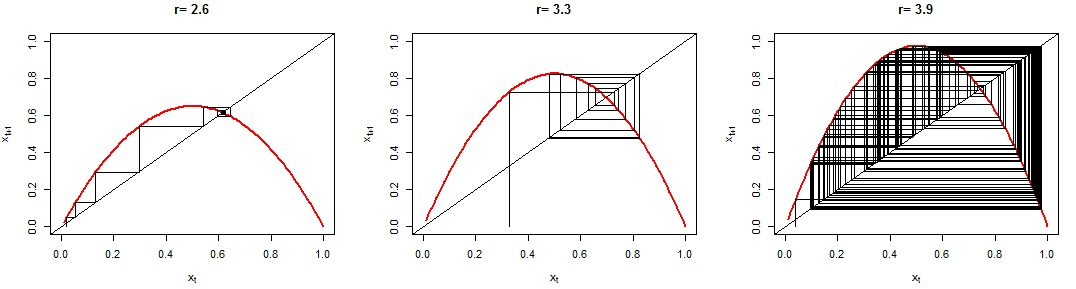8. (Remember to dev.off() before you continue.) Second, for some values of r, the logistic map shows sensitive dependence on initial conditions. For example, let’s see what happens for two different growth rates (r=3 and r=3.9) when we start one iteration with an x[n]  of 0.5 COLORED BLACK, and another one with an x[n] of 0.5001 COLORED RED. It’s a small, small difference that can lead to big, BIG variations in the orbits. In the r=3 case, the chain produced by the logistic map with x[n] of 0.5 (in black) is IDENTICAL to the chain produced by the logistic map with x[n] of 0.5001 (in red). That’s why you can’t see the black… the values are the same! But for the r=3.9 case, the chain produced by the logistic map with x[n] of 0.5 (in black) RAPIDLY DIVERGES from the chain produced by the logistic map with x[n] of 0.5001 (in red). They are very different, despite a very tiny difference in initial conditions! The logistic map for r=3.9 shows a very sensitive dependence on initial conditions

```par(mfrow=c(2,1))
first <- logistic.map(3,.5,120,100)
second <- logistic.map(3,.5001,120,100)
plot(1:length(first),first,type="l",main="r=3 is not sensitive to initial conditions")
lines(1:length(second),second,type="l",col="red")
first <- logistic.map(3.9,.5,120,100)
second <- logistic.map(3.9,.5001,120,100)
plot(1:length(first),first,type="l",main="but r=3.9 is EXTREMELY sensitive")
lines(1:length(second),second,type="l",col="red")

```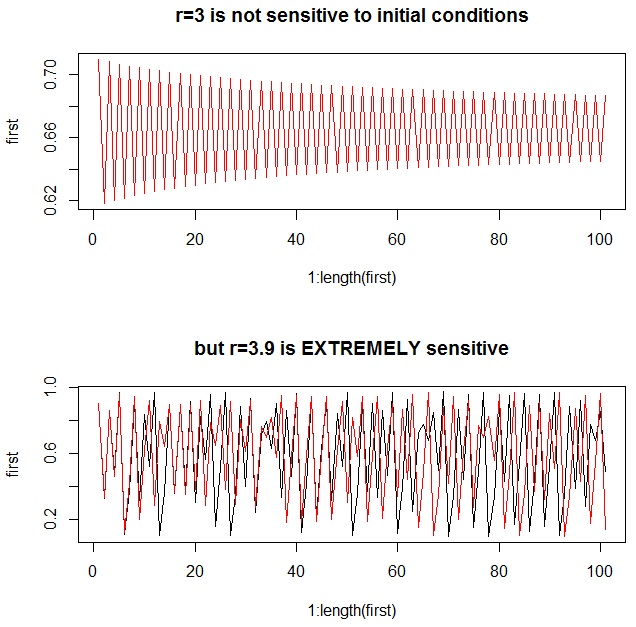9. For any chain, we can determine just how sensitive the logistic map is to initial conditions by looking at the Lyapunov exponent. Very simplistically, if the Lyapunov exponent is negative, the chain will converge to one or more fixed points for that value of r. If the Lyapunov exponent is positive, the chain will demonstrate deterministic chaos for that value of r. If the Lyapunov exponent is zero, there is a bifurcation: a 1-cycle is doubling to a 2-cycle, a 2-cycle is doubling to a 4-cycle, or so forth. The top chart shows an approximation of the Lyapunov exponent based on the first 500 iterations (ideally, you’d use an infinite number, but that would eat up too much computing time), and the bottom chart shows a bifurcation diagramYou’ll notice that the Lyapunov exponents are zero where a bifurcation occurs. To interpret the bifurcation diagram, just remember that each vertical slice through it represents the results of ONE COMPLETELY CONVERGED CHAIN from the logistic map. So it shows the results from many, many, many completely converged chains – and provides an excellent way for us to look at the behavior of MANY different types of populations in just one chart:

```n <- 400
XI <- lya <- 0
x <- seq(0,4,0.01)
for (i in 1:n) {
xi <- logistic.map(x[i],.01,500,500)
XI <- rbind(XI,xi)
}
for (i in 1:length(x)) {
lya[i] <- sum(log(abs(x[i]-(2*x[i]*XI[i,]))))/length(x)
}
plot(x,lya,ylim=c(-4,1),xlim=c(0,4),type="l",main="Lyapunov Exponents for Logistic Map")
abline(h=0, lwd=2, col="red")
# next 3 lines from http://www.magesblog.com/2012/03/logistic-map-feigenbaum-diagram.html:
my.r <- seq(0, 4, by=0.003)
Orbit <- sapply(my.r, logistic.map, x=0.1, N=1000, M=300)
r <- sort(rep(my.r, 301))

par(mfrow=c(2,1))
plot(x,lya,ylim=c(-5,1),xlim=c(0,4),type="l",main="Lyapunov Exponents for Logistic Map")
abline(h=0, col="red", lwd=2)
abline(v=3, col="blue", lwd=2)
plot(r, Orbit, pch=".", cex=0.5, main="Bifurcation Diagram for r=0 to r=4 Logistic Maps")
abline(v=3, col="blue", lwd=2)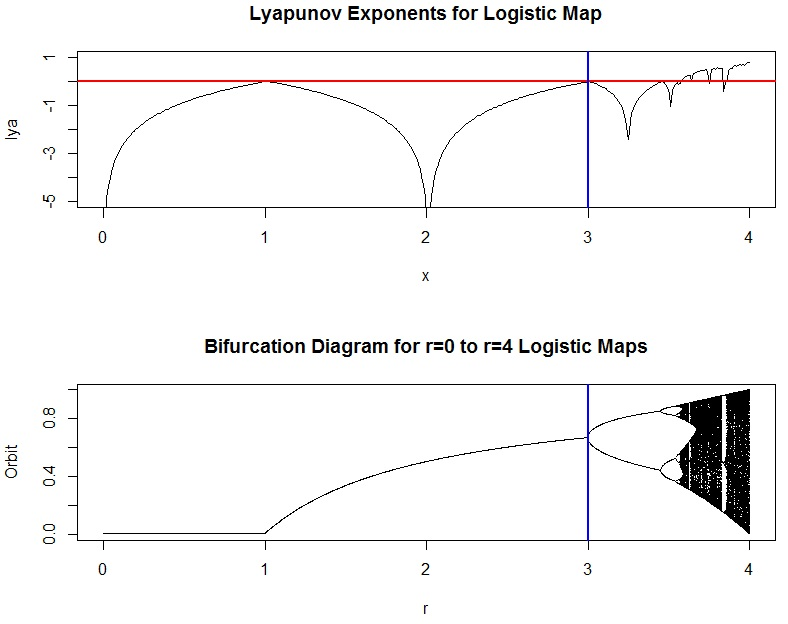```

10. Notice that in the bifurcation diagram, we can easily see that when r is between 0 and 1, the population converges to extinction. This makes sense, because the growth rate is smaller than what’s required to sustain the size of the population. You might like to zoom in, though, and see what the orbits look like for some smaller portions of the diagram. Here’s how you can do it (but be sure to refresh your graphics window with dev.off() before you try it). Try changing the plot character (pch) too, or maybe the size of the characters with cex=0.2 or cex=0.5 in the last line:

```# adapted from http://www.magesblog.com/2012/03/logistic-map-feigenbaum-diagram.html:
my.r <- seq(3.5, 4, by=0.003)
Orbit <- sapply(my.r, logistic.map, x=0.1, N=1000, M=300)
multiplier <- length(Orbit)/length(my.r)
r <- sort(rep(my.r, multiplier))
plot(r, Orbit, pch=".")```That’s it!

Find out more information on these other web pages, which are listed in order of difficulty level:

## A 15-Week Intro Statistics Course Featuring R

Do you teach introductory statistics or data science? Need some help planning your fall class?

I apply the 10 Principles of Burning Man in the design and conduct of all my undergraduate and graduate-level courses, including my introductory statistics class (which has a heavy focus on R and data science) at JMU. This means that I consider learning to be emergent, and as a result, it often doesn’t follow a prescribed path of achieving specified learning objectives. However, in certain courses, I still feel like it’s important to provide a general structure to help guide the way! This also helps the students get a sense of our general trajectory over the course of the semester, and do readings in advance if they’re ready.

Since several people have asked for a copy, here is the SYLLABUS that I use for my 15-week class (that also uses the “informal” TEXTBOOK I wrote this past spring). We meet twice a week for an hour and 15 minutes each session. The class is designed for undergraduate sophomores, but there are always students from all levels enrolled. The course is intended to provide an introduction to (frequentist) statistical thinking, but with an applied focus that has practical data analysis at its core.

My goal is simple. At the end of the semester, I want students to be able to:

Please let me know if this syllabus is helpful to you! I’ll be posting my intensive (5-session) version of this tomorrow or the next day.

Feel free to join our class Facebook group at https://www.facebook.com/groups/262216220608559/ if you want to play along at home.

## A Simple Intro to Bayesian Change Point Analysis

The purpose of this post is to demonstrate change point analysis by stepping through an example of the technique in R presented in Rizzo’s excellent, comprehensive, and very mathy book, Statistical Computing with R, and then showing alternative ways to process this data using the changepoint and bcp packages. Much of the commentary is simplified, and that’s on purpose: I want to make this introduction accessible if you’re just learning the method. (Most of the code is straight from Rizzo who provides a much more in-depth treatment of the technique. I’ve added comments in the code to make it easier for me to follow, and that’s about it.)

The idea itself is simple: you have a sample of observations from a Poisson (counting) process (where events occur randomly over a period of time). You probably have a chart that shows time on the horizontal axis, and how many events occurred on the vertical axis. You suspect that the rate at which events occur has changed somewhere over that range of time… either the event is increasing in frequency, or it’s slowing down — but you want to know with a little more certainty. (Alternatively, you could check to see if the variance has changed, which would be useful for process improvement work in Six Sigma projects.)

You want to estimate the rate at which events occur BEFORE the shift (mu), the rate at which events occur AFTER the shift (lambda), and the time when the shift happens (k). To do it, you can apply a Markov Chain Monte Carlo (MCMC) sampling approach to estimate the population parameters at each possible k, from the beginning of your data set to the end of it. The values you get at each time step will be dependent only on the values you computed at the previous timestep (that’s where the Markov Chain part of this problem comes in). There are lots of different ways to hop around the parameter space, and each hopping strategy has a fancy name (e.g. Metropolis-Hastings, Gibbs, “reversible jump”).

In one example, Rizzo (p. 271-277) uses a Markov Chain Monte Carlo (MCMC) method that applies a Gibbs sampler to do the hopping – with the goal of figuring out the change point in number of coal mine disasters from 1851 to 1962. (Looking at a plot of the frequency over time, it appears that the rate of coal mining disasters decreased… but did it really? And if so, when? That’s the point of her example.) She gets the coal mining data from the boot package. Here’s how to get it, and what it looks like:

```library(boot)
data(coal)
y <- tabulate(floor(coal[]))
y <- y[1851:length(y)]
barplot(y,xlab="years", ylab="frequency of disasters")```First, we initialize all of the data structures we’ll need to use:

```# initialization
n <- length(y) # number of data elements to process
m <- 1000 # target length of the chain
L <- numeric(n) # likelihood fxn has one slot per year
k <- sample(1:n,1) # pick 1 random year to start at
mu <- 1
lambda <- 1
b1 <- 1
b2 <- 1
# now set up blank 1000 element arrays for mu, lambda, and k
mu <- lambda <- k <- numeric(m)```

Here are the models for prior (hypothesized) distributions that she uses, based on the Gibbs sampler approach:

• mu comes from a Gamma distribution with shape parameter of (0.5 + the sum of all your frequencies UP TO the point in time, k, you’re currently at) and a rate of (k + b1)
• lambda comes from a Gamma distribution with shape parameter of (0.5 + the sum of all your frequencies AFTER the point in time, k, you’re currently at) and a rate of (n – k + b1) where n is the number of the year you’re currently processing
• b1 comes from a Gamma distribution with a shape parameter of 0.5 and a rate of (mu + 1)
• b2 comes from a Gamma distribution with a shape parameter of 0.5 and a rate of (lambda + 1)
• a likelihood function L is also provided, and is a function of k, mu, lambda, and the sum of all the frequencies up until that point in time, k

At each iteration, you pick a value of k to represent a point in time where a change might have occurred. You slice your data into two chunks: the chunk that happened BEFORE this point in time, and the chunk that happened AFTER this point in time. Using your data, you apply a Poisson Process with a (Hypothesized) Gamma Distributed Rate as your model. This is a pretty common model for this particular type of problem. It’s like randomly cutting a deck of cards and taking the average of the values in each of the two cuts… then doing the same thing again… a thousand times. Here is Rizzo’s (commented) code:

```# start at 2, so you can use initialization values as seeds
# and go through this process once for each of your m iterations
for (i in 2:m) {
kt <- k[i-1] # start w/random year from initialization
# set your shape parameter to pick mu from, based on the characteristics
# of the early ("before") chunk of your data
r <- .5 + sum(y[1:kt])
# now use it to pick mu
mu[i] <- rgamma(1,shape=r,rate=kt+b1)
# if you're at the end of the time periods, set your shape parameter
# to 0.5 + the sum of all the frequencies, otherwise, just set the shape
# parameter that you will use to pick lambda based on the later ("after")
# chunk of your data
if (kt+1 > n) r <- 0.5 + sum(y) else r <- 0.5 + sum(y[(kt+1):n])
lambda[i] <- rgamma(1,shape=r,rate=n-kt+b2)
# now use the mu and lambda values that you got to set b1 and b2 for next iteration
b1 <- rgamma(1,shape=.5,rate=mu[i]+1)
b2 <- rgamma(1,shape=.5,rate=lambda[i]+1)
# for each year, find value of LIKELIHOOD function which you will
# then use to determine what year to hop to next
for (j in 1:n) {
L[j] <- exp((lambda[i]-mu[i])*j) * (mu[i]/lambda[i])^sum(y[1:j])
}
L <- L/sum(L)
# determine which year to hop to next
k[i] <- sample(1:n,prob=L,size=1)
}```

Knowing the distributions of mu, lambda, and k from hopping around our data will help us estimate values for the true population parameters. At the end of the simulation, we have an array of 1000 values of k, an array of 1000 values of mu, and an array of 1000 values of lambda — we use these to estimate the real values of the population parameters. Typically, algorithms that do this automatically throw out a whole bunch of them in the beginning (the “burn-in” period) — Rizzo tosses out 200 observations — even though some statisticians (e.g. Geyer) say that the burn-in period is unnecessary:

```> b <- 201 # treats time until the 200th iteration as "burn-in"
> mean(k[b:m])
 39.765
> mean(lambda[b:m])
 0.9326437
> mean(mu[b:m])
 3.146413```

The change point happened between the 39th and 40th observations, the arrival rate before the change point was 3.14 arrivals per unit time, and the rate after the change point was 0.93 arrivals per unit time. (Cool!)
After I went through this example, I discovered the changepoint package, which let me run through a similar process in just a few lines of code. Fortunately, the results were very similar! I chose the “AMOC” method which stands for “at most one change”. Other methods are available which can help identify more than one change point (PELT, BinSeg, and SegNeigh – although I got an error message every time I attempted that last method).

```> results <- cpt.mean(y,method="AMOC")
> cpts(results)
cpt
36
> param.est(results)
\$mean
 3.2500000 0.9736842
> plot(results,cpt.col="blue",xlab="Index",cpt.width=4)```I decided to explore a little further and found even MORE change point analysis packages! So I tried this example using bcp (which I presume stands for “Bayesian Change Point”) and voila… the output looks very similar to each of the previous two methods!!!):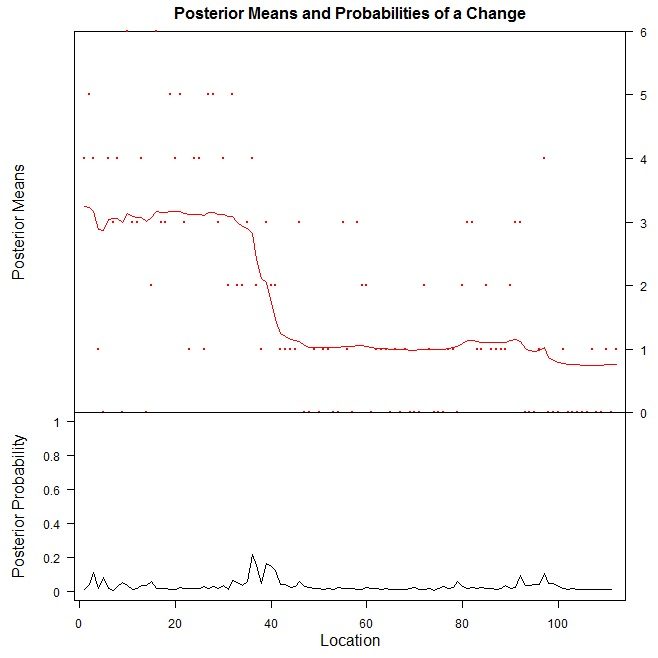It’s at this point that the HARD part of the data science project would begin… WHY? Why does it look like the rate of coal mining accidents decreased suddenly? Was there a change in policy or regulatory requirements in Australia, where this data was collected? Was there some sort of mass exodus away from working in the mines, and so there’s a covariate in the number of opportunities for a mining disaster to occur? Don’t know… the original paper from 1979 doesn’t reveal the true story behind the data.

There are also additional resources on R Bloggers that discuss change point analysis:

(Note: If I’ve missed anything, or haven’t explained anything right, please provide corrections and further insights in the comments! Thank you.

## Sampling Distributions and Central Limit Theorem in R

The Central Limit Theorem (CLT), and the concept of the sampling distribution, are critical for understanding why statistical inference works. There are at least a handful of problems that require you to invoke the Central Limit Theorem on every ASQ Certified Six Sigma Black Belt (CSSBB) exam. The CLT says that if you take many repeated samples from a population, and calculate the averages or sum of each one, the collection of those averages will be normally distributed… and it doesn’t matter what the shape of the source distribution is! (Caveat: so long as the data comes from a distribution with finite variance… so that means the Cauchy distribution doesn’t count.)

I wrote some R code to help illustrate this principle for my students. This code allows you to choose a sample size (n), a source distribution, and parameters for that source distribution, and generate a plot of the sampling distributions of the mean, sum, and variance. (Note: the sampling distribution for the variance is a Chi-square distribution — if your source distribution is normal!)

```sdm.sim <- function(n,src.dist=NULL,param1=NULL,param2=NULL) {
r <- 10000  # Number of replications/samples - DO NOT ADJUST
# This produces a matrix of observations with
# n columns and r rows. Each row is one sample:
my.samples <- switch(src.dist,
"E" = matrix(rexp(n*r,param1),r),
"N" = matrix(rnorm(n*r,param1,param2),r),
"U" = matrix(runif(n*r,param1,param2),r),
"P" = matrix(rpois(n*r,param1),r),
"B" = matrix(rbinom(n*r,param1,param2),r),
"G" = matrix(rgamma(n*r,param1,param2),r),
"X" = matrix(rchisq(n*r,param1),r),
"T" = matrix(rt(n*r,param1),r))
all.sample.sums <- apply(my.samples,1,sum)
all.sample.means <- apply(my.samples,1,mean)
all.sample.vars <- apply(my.samples,1,var)
par(mfrow=c(2,2))
hist(my.samples[1,],col="gray",main="Distribution of One Sample")
hist(all.sample.sums,col="gray",main="Sampling Distribution\nof
the Sum")
hist(all.sample.means,col="gray",main="Sampling Distribution\nof the Mean")
hist(all.sample.vars,col="gray",main="Sampling Distribution\nof
the Variance")
}```

There are 8 population distributions to choose from: exponential (E), normal (N), uniform (U), Poisson (P), binomial (B), gamma (G), Chi-Square (X), and the Student’s t distribution (T). Note also that you have to provide either one or two parameters, depending upon what distribution you are selecting. For example, a normal distribution requires that you specify the mean and standard deviation to describe where it’s centered, and how fat or thin it is (that’s two parameters). A Chi-square distribution requires that you specify the degrees of freedom (that’s only one parameter). You can find out exactly what distributions require what parameters by going here: http://en.wikibooks.org/wiki/R_Programming/Probability_Distributions.

Here is an example that draws from an exponential distribution with a mean of 1/1 (you specify the number you want in the denominator of the mean):

`sdm.sim(50,src.dist="E",param1=1)`

The code above produces this sequence of plots:You aren’t allowed to change the number of replications in this simulation because of the nature of the sampling distribution: it’s a theoretical model that describes the distribution of statistics from an infinite number of samples. As a result, if you increase the number of replications, you’ll see the mean of the sampling distribution bounce around until it converges on the mean of the population. This is just an artifact of the simulation process: it’s not a characteristic of the sampling distribution, because to be a sampling distribution, you’ve got to have an infinite number of samples. Watkins et al. have a great description of this effect that all statistics instructors should be aware of. I chose 10,000 for the number of replications because 1) it’s close enough to infinity to ensure that the mean of the sampling distribution is the same as the mean of the population, but 2) it’s far enough away from infinity to not crash your computer, even if you only have 4GB or 8GB of memory.

Here are some more examples to try. You can see that as you increase your sample size (n), the shapes of the sampling distributions become more and more normal, and the variance decreases, constraining your estimates of the population parameters more and more.

```sdm.sim(10,src.dist="E",1)
sdm.sim(50,src.dist="E",1)
sdm.sim(100,src.dist="E",1)
sdm.sim(10,src.dist="X",14)
sdm.sim(50,src.dist="X",14)
sdm.sim(100,src.dist="X",14)
sdm.sim(10,src.dist="N",param1=20,param2=3)
sdm.sim(50,src.dist="N",param1=20,param2=3)
sdm.sim(100,src.dist="N",param1=20,param2=3)
sdm.sim(10,src.dist="G",param1=5,param2=5)
sdm.sim(50,src.dist="G",param1=5,param2=5)
sdm.sim(100,src.dist="G",param1=5,param2=5)```

## One-proportion z test in R

For quite a while, I’ve been confused by the behavior of the prop.test function in R: 1) the p-values always seem to be slightly off, and 2) the confidence intervals never seem to be symmetric around my estimate of the population proportion. Today, I decided I was going to figure out what was up… and whether I should trust my own analytical calculations for this test or what I get in R. I started by checking R Bloggers to see if anyone had explored this problem, but I only found one post about the two proportion z-test, and found none focusing on the one proportion z-test.

Here’s the essence of the test: you observe x subjects out of a sample size of n observations. Perhaps you just want to create a confidence interval to get a sense of where the true population proportion lies. Or maybe, you’d like to test the null hypothesis that the true population proportion is some value p0, against the alternative that it is really greater than, less than, or not equal to that value.

To do this, you can use the normal approximation to the binomial distribution to calculate a test statistic z. The estimated population proportion, p-hat, is just x/n. You have to find the standard error (SE) of your estimate as well to get the value of z: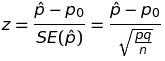The confidence interval is constructed from the estimated population proportion, and the margin of error, which is determined by the standard error of the estimate and a scaling factor (z*) that establishes the width of the confidence interval: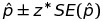Here’s an example. Let’s say I know that nationally, 90% of home buyers would select a home with energy efficient features, even paying a premium of 2-3%, to reduce their utility costs long term. As the hypothetical president of an engineering firm that installs these energy efficient features, I want to know if less than 90% of buyers in my local area feel this way (because if so, I might want to launch an education campaign to help my business). I randomly sample 360 potential buyers and find that 312 of them would indeed select a home with energy efficient features.

I want to test my sample against the null hypothesis that the true population proportion is 0.90, with the alternative that the proportion is less than 0.90. I use a estimated population proportion (p-hat) of 312/360=0.867, and using the equations above, find that my test statistic z turns out to be -2.108, with a corresponding p-value of 0.0175. I reject the null hypothesis that the true population proportion is 0.90 in favor of the alternative, and start making plans to launch my education program.

(I don’t get the same values when I use prop.test. But later in this post, I’ll show you why I shouldn’t have expected that in the first place!!)

I wrote an R function to automate my z test (and also, to double check my calculations):

```z.test <- function(x,n,p=NULL,conf.level=0.95,alternative="less") {
ts.z <- NULL
cint <- NULL
p.val <- NULL
phat <- x/n
qhat <- 1 - phat
# If you have p0 from the population or H0, use it.
# Otherwise, use phat and qhat to find SE.phat:
if(length(p) > 0) {
q <- 1-p
SE.phat <- sqrt((p*q)/n)
ts.z <- (phat - p)/SE.phat
p.val <- pnorm(ts.z)
if(alternative=="two.sided") {
p.val <- p.val * 2
}
if(alternative=="greater") {
p.val <- 1 - p.val
}
} else {
# If all you have is your sample, use phat to find
# SE.phat, and don't run the hypothesis test:
SE.phat <- sqrt((phat*qhat)/n)
}
cint <- phat + c(
-1*((qnorm(((1 - conf.level)/2) + conf.level))*SE.phat),
((qnorm(((1 - conf.level)/2) + conf.level))*SE.phat) )
return(list(estimate=phat,ts.z=ts.z,p.val=p.val,cint=cint))
}```

When I run it on my example above, I get what I expect:

```> z.test(312,360,p=0.9)
\$estimate
 0.867

\$ts.z
 -2.11

\$p.val
 0.0175

\$cint
 0.836 0.898

```

But when I run prop.test, this isn’t what I see at all!

```> prop.test(312,360,p=0.9)

1-sample proportions test with continuity correction

data: 312 out of 360, null probability 0.9
X-squared = 4.08, df = 1, p-value = 0.04335
alternative hypothesis: true p is not equal to 0.9
95 percent confidence interval:
0.826 0.899
sample estimates:
p
0.867

```

Why are there differences? Here’s what I found out:

• The prop.test function doesn’t even do a z test. It does a Chi-square test, based on there being one categorical variable with two states (success and failure)! Which I should have noticed immediately from the line that starts with “X-squared” 🙂
• The Yates continuity correction, which adjusts for the differences that come from using a normal approximation to the binomial distribution, is also automatically applied. This removes 0.5/n from the lower limit of the confidence interval, and adds 0.5/n to the upper limit.
• Most notably, I was really disturbed to see that the confidence interval given by prop.test wasn’t even centered around the sample estimate, p-hat. Oh no! But again, this is no concern, since prop.test uses the Wilson score interval to construct the confidence interval. This results in an asymmetric, but presumably more accurate (with respect to the real population) confidence interval.

Moral of the story? There are many ways to test an observed proportion against a standard, target, or recommended value. If you’re doing research, and especially if you’re using R, be sure to know what’s going on under the hood before you report your results.

(Other moral? Try binom.test.)